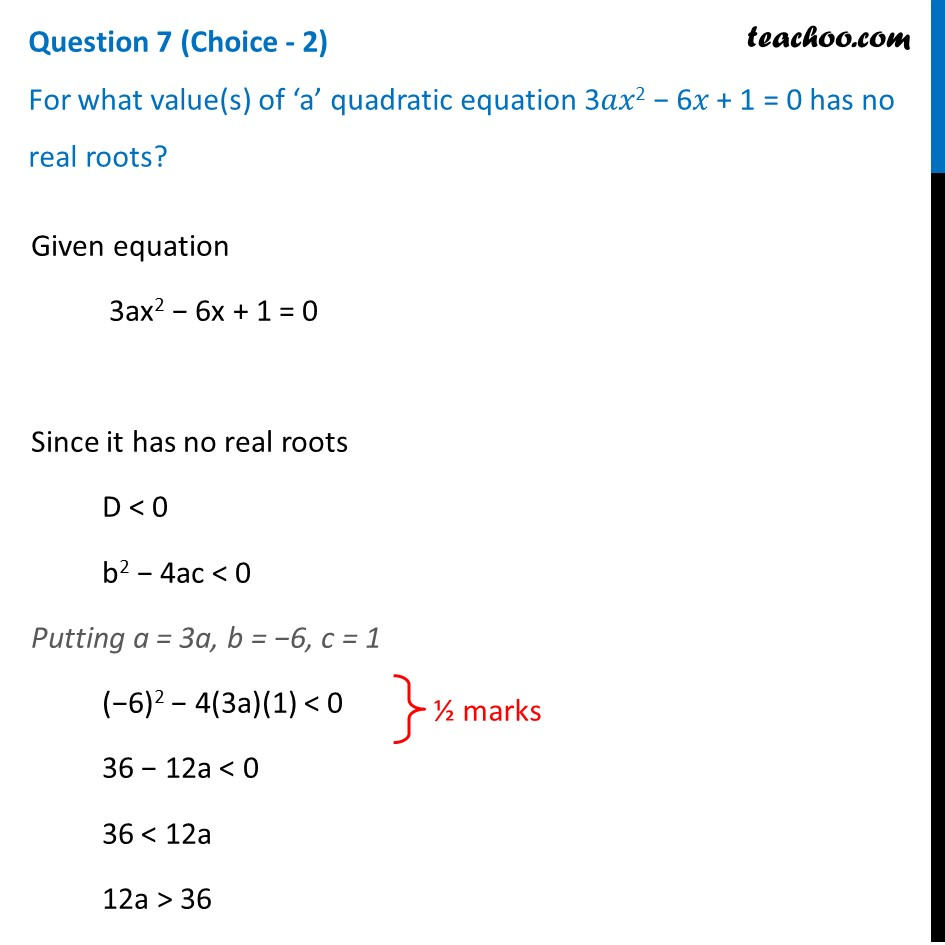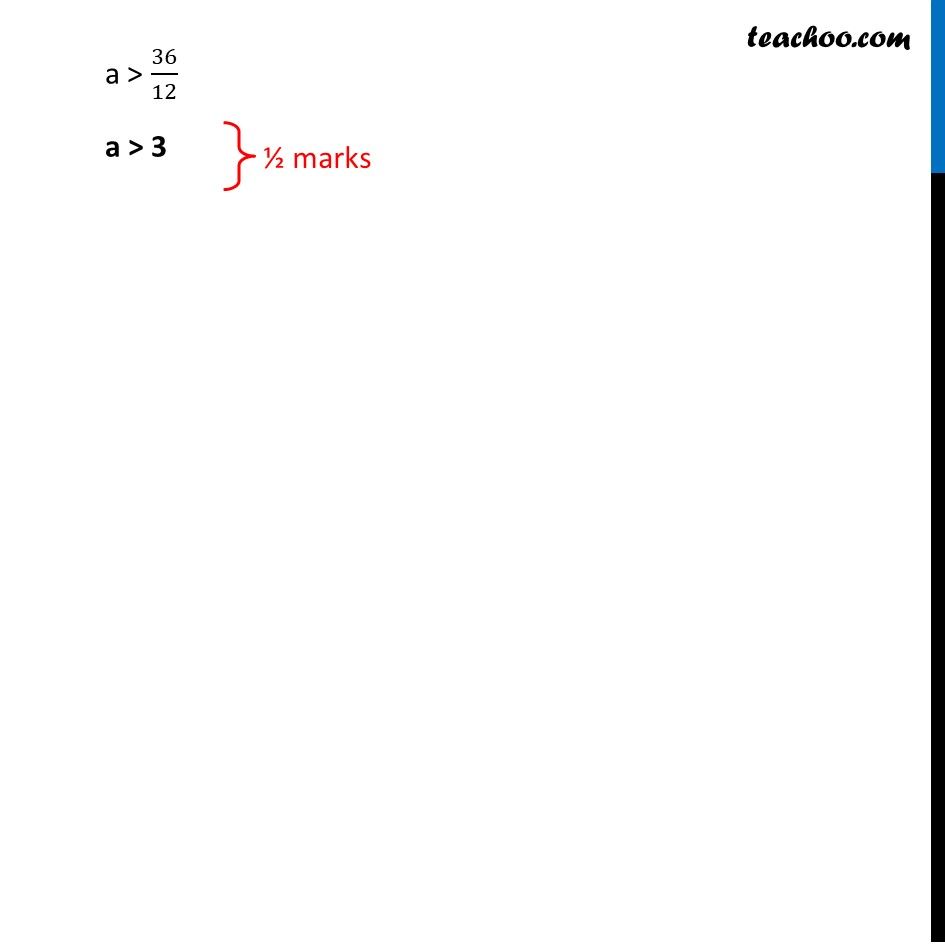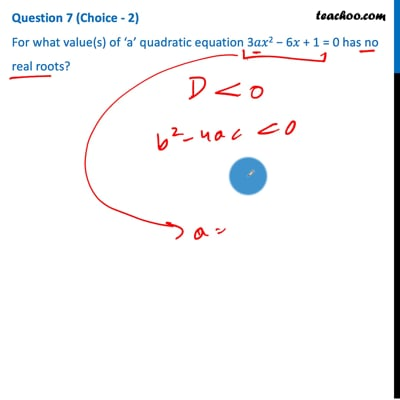CBSE Class 10 Sample Paper for 2021 Boards - Maths Standard

Class 10
Solutions of Sample Papers for Class 10 Boards

## For what value(s) of ‘a’ quadratic equation 3𝑎𝑥 2 − 6𝑥 + 1 = 0 has no real roots?This video is only available for Teachoo black users

Introducing your new favourite teacher - Teachoo Black, at only ₹83 per month

### Transcript

Question 7 (Choice - 2) For what value(s) of ‘a’ quadratic equation 3𝑎𝑥2 − 6𝑥 + 1 = 0 has no real roots? Given equation 3ax2 − 6x + 1 = 0 Since it has no real roots D < 0 b2 − 4ac < 0 Putting a = 3a, b = −6, c = 1 (−6)2 − 4(3a)(1) < 0 36 − 12a < 0 36 < 12a 12a > 36 a > 36/12 a > 3